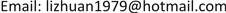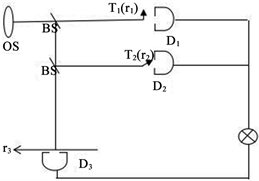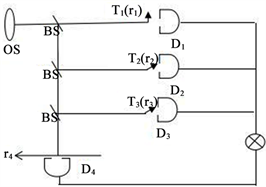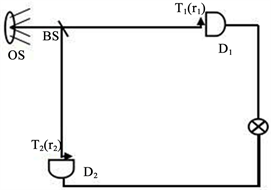﻿ 基于高阶关联成像的拓展研究 Extended Research Based on High-Order Correlation Imaging

Applied Physics
Vol. 08  No. 10 ( 2018 ), Article ID: 27117 , 5 pages
10.12677/APP.2018.810054

Extended Research Based on High-Order Correlation Imaging

Zhuan Li, Chunmei Liu, Junling Wang

Basic Education Department, Army Academy of Armored Forces, BeijingReceived: Sep. 30th, 2018; accepted: Oct. 8th, 2018; published: Oct. 15th, 2018ABSTRACT

Using the method of high-order correlation imaging, the image of multiple scattered objects in the same place is achieved, and the ghost imaging puzzle is realized. Bucket detection is taken to determine the similarity between the detected object and the reference object by measuring the intensity correlation between the two detectors, which provides a theoretical way of realizing object recognition.

Keywords:High-Order Correlation Imaging, Bucket Detection, Jigsaw, Object RecognitionCopyright © 2018 by authors and Hans Publishers Inc.1. 引言

2. 鬼成像拼图

2.1. 两个物体的鬼成像拼图

$\begin{array}{l}{I}_{j}=\int {T}_{j}^{2}\left({\stackrel{⇀}{r}}_{j}\right){E}_{j}^{*}\left({\stackrel{⇀}{r}}_{j}\right){E}_{j}\left({\stackrel{⇀}{r}}_{j}\right)\text{\hspace{0.17em}}\text{d}{\stackrel{⇀}{r}}_{j},\text{\hspace{0.17em}}j=1,2\\ {I}_{3}\left({\stackrel{⇀}{r}}_{3}\right)={E}_{3}^{*}\left({\stackrel{⇀}{r}}_{3}\right){E}_{3}\left({\stackrel{⇀}{r}}_{3}\right),\end{array}$ (1)

${G}^{\left(3,3\right)}\left({\stackrel{⇀}{r}}_{3}\right)=〈{I}_{1}{I}_{2}{I}_{3}\left({\stackrel{⇀}{r}}_{3}\right)〉\text{=}〈\int {T}_{1}^{2}\left({\stackrel{⇀}{r}}_{1}\right){T}_{2}^{2}\left({\stackrel{⇀}{r}}_{2}\right){E}_{1}^{*}\left({\stackrel{⇀}{r}}_{1}\right){E}_{2}^{*}\left({\stackrel{⇀}{r}}_{2}\right){E}_{3}^{*}\left({\stackrel{⇀}{r}}_{3}\right){E}_{3}\left({\stackrel{⇀}{r}}_{3}\right){E}_{2}\left({\stackrel{⇀}{r}}_{2}\right){E}_{1}\left({\stackrel{⇀}{r}}_{1}\right)\text{d}{\stackrel{⇀}{r}}_{1}\text{d}{\stackrel{⇀}{r}}_{2}〉,$ (2)

${G}^{\left(3,3\right)}\left({\stackrel{⇀}{r}}_{3}\right)=\int {T}_{1}^{2}\left({\stackrel{⇀}{r}}_{1}\right){T}_{2}^{2}\left({\stackrel{⇀}{r}}_{2}\right){G}^{\left(3,3\right)}\left({\stackrel{⇀}{r}}_{1},{\stackrel{⇀}{r}}_{2},{\stackrel{⇀}{r}}_{3}\right)\text{d}{\stackrel{⇀}{r}}_{1}\text{d}{\stackrel{⇀}{r}}_{2},$ (3)

${G}^{\left(3,3\right)}\left({\stackrel{⇀}{r}}_{1},{\stackrel{⇀}{r}}_{2},{\stackrel{⇀}{r}}_{3}\right)=〈{E}_{1}^{*}\left({\stackrel{⇀}{r}}_{1}\right){E}_{2}^{*}\left({\stackrel{⇀}{r}}_{2}\right){E}_{3}^{*}\left({\stackrel{⇀}{r}}_{3}\right){E}_{3}\left({\stackrel{⇀}{r}}_{3}\right){E}_{2}\left({\stackrel{⇀}{r}}_{2}\right){E}_{1}\left({\stackrel{⇀}{r}}_{1}\right)〉.$ (4)

$\begin{array}{c}{G}^{\left(3,3\right)}\left({\stackrel{⇀}{r}}_{1},{\stackrel{⇀}{r}}_{2},{\stackrel{⇀}{r}}_{3}\right)=〈{I}_{1}\left({\stackrel{⇀}{r}}_{1}\right)〉〈{I}_{2}\left({\stackrel{⇀}{r}}_{2}\right)〉〈{I}_{3}\left({\stackrel{⇀}{r}}_{3}\right)〉+〈{I}_{1}\left({\stackrel{⇀}{r}}_{1}\right)〉{|〈{E}_{2}^{*}\left({\stackrel{⇀}{r}}_{2}\right){E}_{3}\left({\stackrel{⇀}{r}}_{3}\right)〉|}^{2}+〈{I}_{2}\left({\stackrel{⇀}{r}}_{2}\right)〉{|〈{E}_{1}^{*}\left({\stackrel{⇀}{r}}_{1}\right){E}_{3}\left({\stackrel{⇀}{r}}_{3}\right)〉|}^{2}\\ +〈{I}_{3}\left({\stackrel{⇀}{r}}_{3}\right)〉{|〈{E}_{1}^{*}\left({\stackrel{⇀}{r}}_{1}\right){E}_{2}\left({\stackrel{⇀}{r}}_{2}\right)〉|}^{2}+\left[〈{E}_{1}^{*}\left({\stackrel{⇀}{r}}_{1}\right){E}_{2}\left({\stackrel{⇀}{r}}_{2}\right)〉〈{E}_{2}^{*}\left({\stackrel{⇀}{r}}_{2}\right){E}_{3}\left({\stackrel{⇀}{r}}_{3}\right)〉〈{E}_{3}^{*}\left({\stackrel{⇀}{r}}_{3}\right){E}_{1}\left({\stackrel{⇀}{r}}_{1}\right)〉+c.c\right],\end{array}$ (5)Figure 1. The ghost imaging puzzle for two objects

$〈{E}_{s}^{*}\left({\stackrel{⇀}{r}}_{s}\right){E}_{s}\left({\stackrel{⇀}{r}}_{s}^{\text{'}}\right)〉={I}_{s}\delta \left({\stackrel{⇀}{r}}_{s}-{\stackrel{⇀}{r}}_{s}^{\text{'}}\right),$ (6)

$〈{E}_{i}^{*}\left({\stackrel{⇀}{r}}_{i}\right){E}_{j}\left({\stackrel{⇀}{r}}_{j}\right)〉=I\delta \left({\stackrel{⇀}{r}}_{i}-{\stackrel{⇀}{r}}_{j}\right).$ (7)

$\begin{array}{c}{G}^{\left(3,3\right)}\left({\stackrel{⇀}{r}}_{3}\right)={I}^{3}\int {T}_{1}^{2}\left({\stackrel{⇀}{r}}_{1}\right){T}_{2}^{2}\left({\stackrel{⇀}{r}}_{2}\right)\text{\hspace{0.17em}}\text{d}{\stackrel{⇀}{r}}_{1}\text{d}{\stackrel{⇀}{r}}_{2}+{I}^{3}{T}_{2}^{2}\left({\stackrel{⇀}{r}}_{3}\right)\int {T}_{1}^{2}\left({\stackrel{⇀}{r}}_{1}\right)\text{\hspace{0.17em}}\text{d}{\stackrel{⇀}{r}}_{1}\\ +{I}^{3}{T}_{1}^{2}\left({\stackrel{⇀}{r}}_{3}\right)\int {T}_{2}^{2}\left({\stackrel{⇀}{r}}_{2}\right)\text{\hspace{0.17em}}\text{d}{\stackrel{⇀}{r}}_{2}+{I}^{3}\int {T}_{1}^{2}\left({\stackrel{⇀}{r}}_{1}\right){T}_{2}^{2}\left({\stackrel{⇀}{r}}_{1}\right)\text{\hspace{0.17em}}\text{d}{\stackrel{⇀}{r}}_{1}+2{I}^{3}{T}_{1}^{2}\left({\stackrel{⇀}{r}}_{3}\right){T}_{2}^{2}\left({\stackrel{⇀}{r}}_{3}\right),\end{array}$ (8)

${G}^{\left(3,3\right)}\left({\stackrel{⇀}{r}}_{3}\right)={I}^{3}\left[{S}_{1}{S}_{2}+{S}_{12}+{S}_{2}{T}_{1}^{2}\left({\stackrel{⇀}{r}}_{3}\right)+{S}_{1}{T}_{2}^{2}\left({\stackrel{⇀}{r}}_{3}\right)+2{T}_{1}^{2}\left({\stackrel{⇀}{r}}_{3}\right){T}_{2}^{2}\left({\stackrel{⇀}{r}}_{3}\right)\right],$ (9)

2.2. 三个物体的鬼成像拼图

${G}^{\left(4,4\right)}\left({\stackrel{⇀}{r}}_{4}\right)={I}^{4}\left[\begin{array}{l}{S}_{1}{S}_{2}{S}_{3}+{S}_{1}{S}_{23}+{S}_{2}{S}_{13}+{S}_{3}{S}_{12}+2{S}_{123}+\left({S}_{23}+{S}_{2}{S}_{3}\right){T}_{1}^{2}\left({\stackrel{⇀}{r}}_{4}\right)\\ +\left({S}_{13}+{S}_{1}{S}_{3}\right){T}_{2}^{2}\left({\stackrel{⇀}{r}}_{4}\right)+\left({S}_{12}+{S}_{1}{S}_{2}\right){T}_{3}^{2}\left({\stackrel{⇀}{r}}_{4}\right)+2{S}_{1}{T}_{2}^{2}\left({\stackrel{⇀}{r}}_{4}\right){T}_{3}^{2}\left({\stackrel{⇀}{r}}_{4}\right)\\ +2{S}_{2}{T}_{1}^{2}\left({\stackrel{⇀}{r}}_{4}\right){T}_{3}^{2}\left({\stackrel{⇀}{r}}_{4}\right)+2{S}_{3}{T}_{1}^{2}\left({\stackrel{⇀}{r}}_{4}\right){T}_{2}^{2}\left({\stackrel{⇀}{r}}_{4}\right)\text{+6}{T}_{1}^{2}\left({\stackrel{⇀}{r}}_{4}\right){T}_{2}^{2}\left({\stackrel{⇀}{r}}_{4}\right){T}_{3}^{2}\left({\stackrel{⇀}{r}}_{4}\right)\end{array}\right],$ (10)

${S}_{i}=\int {T}_{i}^{2}\left({\stackrel{⇀}{r}}_{i}\right)\text{\hspace{0.17em}}\text{d}{\stackrel{⇀}{r}}_{i}$ 表示物体 $i\left(i=\text{1},\text{2},\text{3}\right)$ 的面积， ${S}_{ij}=\int {T}_{i}^{2}\left({\stackrel{⇀}{r}}_{i}\right){T}_{j}^{2}\left({\stackrel{⇀}{r}}_{i}\right)\text{\hspace{0.17em}}\text{d}{\stackrel{⇀}{r}}_{i}$ 表示i和j两物体乘积的面积， ${S}_{123}=\int {T}_{1}^{2}\left({\stackrel{⇀}{r}}_{i}\right){T}_{2}^{2}\left({\stackrel{⇀}{r}}_{i}\right){T}_{3}^{2}\left({\stackrel{⇀}{r}}_{i}\right)\text{\hspace{0.17em}}\text{d}\stackrel{⇀}{r},\text{\hspace{0.17em}}i\left(i=\text{1},\text{2},\text{3}\right)$ 表示三物体乘积的面积。

3. 目标识别

$〈{I}_{1}{I}_{2}〉=〈{I}_{1}〉〈{I}_{2}〉+\int {|〈{E}_{1}^{*}\left({\stackrel{⇀}{r}}_{1}\right){E}_{2}\left({\stackrel{⇀}{r}}_{2}\right)〉|}^{2}\text{d}{\stackrel{⇀}{r}}_{1}\text{d}{\stackrel{⇀}{r}}_{2}=〈{I}_{1}〉〈{I}_{2}〉+{I}_{0}^{2}{S}_{12},$ (11)

$〈{I}_{1}〉$$〈{I}_{2}〉$ 分别为两探测器所测得的单路平均强度。 ${S}_{12}$ 表示两物体乘积的面积,当两物体完全相同且放置位置相同时取 ${S}_{12}=1$ ，当两物体完全正交或者完全不同时 ${S}_{12}=0$ ，这种测量方式可以为进行目标识别提供方式方法。

$〈{I}_{1}{I}_{2}〉-〈{I}_{1}〉〈{I}_{2}〉={I}_{0}^{2}{S}_{12}.$ (12)

$0\le {S}_{12}\le 1.$ (13)Figure 2. The ghost imaging puzzle for three objectsFigure 3. The model for the object recognition

4. 小结

Extended Research Based on High-Order Correlation Imaging[J]. 应用物理, 2018, 08(10): 427-431. https://doi.org/10.12677/APP.2018.810054

1. 1. Bennink, R.S., Bentley, S.J. and Boyd, R.W. (2002) Two-Photon Coincidence Imaging with a Classical Source. Physical Review Letters, 89, 113601. https://doi.org/10.1103/PhysRevLett.89.113601

2. 2. 汪凯戈, 曹德忠, 熊俊. 关联光学新进展[J]. 物理, 2008, 37(4): 223-232.

3. 3. Liu, X.F., Li, M.F., Yao, X.R., et al. (2013) High-Visibility Ghost Imaging from Artificially Generated Non-Gaussian Intensity Fluctuations. AIP Advances, 3, 052121. https://doi.org/10.1063/1.4807655

4. 4. 欧丽华. 二阶和三阶关联成像理论研究[D]. 长沙: 湖南师范大学, 2007.

5. 5. Chan, K.W., Sullivan, M.N. and Boyd, R.W. (2009) High-Order Thermal Ghost Imaging. Optics Letters, 34, 3343-3345. https://doi.org/10.1364/OL.34.003343

6. 6. Liu, H.-C. and Xiong, J. (2013) Properties of High-Order Ghost Imaging with Natural Light. Journal of Optical Society of America A, 30, 956-961. https://doi.org/10.1364/JOSAA.30.000956

7. 7. Chen, X.-H., Wu, S.-S., Wu, W., et al. (2014) An Improved Algorithm to Re-duce Noise in High-Order Thermal Ghost Imaging. Journal of Optical Society of America A, 31, 2105-2108. https://doi.org/10.1364/JOSAA.31.002105

8. 8. Cao, D.-Z., Li, Q.-C., Zhuang, X.-C., et al. (2018) Fractional-Order Correlation Imaging with Thermal Light. arxiv:1801.04680v1.

9. 9. Zhang, S.-Z., Song, H.-L., Xiang, X.-Y., et al. (2010) Fast SIFT Algorithm for Object Recognition. Computer Systems & Applications, 19, 82-85.

10. 10. Wang, P., Gao, Y.-H., Li, B., et al. (2012) Technology of Detection and Recognition of Laser Imaging Radar Space Target. Modern Defence Technology, 40, 31-36.